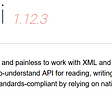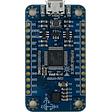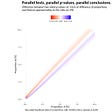# The Two Sum problem

There are many different versions of this very famous problem. I will visit some of the variants of this problem, starting from the very basic one:

## The Two Sum Problem (basic)

Problem Description: Given an array of integers `nums` and an integer `target`, return indices of the two numbers such that they add up to `target`.You may assume that each input would have exactly one solution, and you may not use the same element twice.You can return the answer in any order.

So, obviously, if you are very new to such problem solving, you’ll think of the brute force method where you will have two nested loops, where for every number in the array, you’ll check every other number for a match. That will have a time complexity of O(n²) which is definitely very inefficient.

So, the most efficient way of solving this problem is by using a HashMap. Using every number in the array as the key and their respective indices as the value, we can store all the numbers while simultaneously checking if the complement of that number already exists in the map. If it does, and because we are told that there exists only ONE unique solution, we can simply return the indices from the function.

Here’s my solution in Java:

`    public int[] twoSum(int[] nums, int target) {                HashMap <Integer, Integer> map = new HashMap<>();        int [] result = new int ;                for(int i=0; i<nums.length; i++){            if(map.containsKey(target-nums[i]) && map.get(target-nums[i])!=i)                return new int[] { i, map.get(target - nums[i]) };                        else                 map.put(nums[i], i);          }               throw new IllegalArgumentException("No two sum solution");    }`

Analysis: The time complexity of this solution is O(n) and it only goes through the array once. We could have made the solution simpler by making one loop to fill the map and another loop to find the answer but that will take longer, precisely O(2n). This right here is the most efficient solution to the Two Sum Problem.

## The Two Sum II — Input array is sorted

Now, we can look at a very similar problem, where everything is the same as the one we discussed, only the given array nums[] is pre-sorted. The following is the problem description:

Problem Description: Given an array of integers `numbers` that is already sorted in ascending order, find two numbers such that they add up to a specific `target` number. Return the indices of the two numbers (1-indexed) as an integer array `answer` of size `2`, where `1 <= answer < answer <= numbers.length`. You may assume that each input would have exactly one solution and you may not use the same element twice.

Now, we can easily apply the above solution with a slight change to ensure that our result is 1-indexed and sorted. It solves ht problem and passes all the test cases. However, is it the most efficient? Let’s see, the given array is currently sorted so do we really need to compare every combination of sums of the array? That is too much redundant calculation. Let us instead look at the following approach:

`   public int[] twoSum(int[] numbers, int target) {        int i=0;        int j=numbers.length-1;        while(i<j){            int sum = numbers[i] + numbers [j];                       if(sum < target){                i++;            }            else if(sum > target){                j--;            }            else                return new int[] {i+1, j+1};                        }         throw new IllegalArgumentException("No two sum solution");   }`

Analysis: So, here we take advantage of the fact that the array is already sorted. We start by setting our i to the first element (i.e. smallest) and j to the very last (i.e largest) number in the array. That means if we realize that our current sum is less than the target sum, we know for sure that we need to increase our smaller number, therefore we do i++. On the other hand, if we realize that our current sum is larger than our target, it definitely means our larger number is too big. Therefore, we do a j--. And if we reach the final else statement, it means we have found our match. and because i is ALWAYS less than j, we can simply make an array with i as the 1st and j as the 2nd element and return it. Lastly, an exception is thrown if the program exits from the for-loop without returning from the function as it means no two-sum solution of the given target was found in the array.

## Two Sum III — Data structure design

This one is very similar to The Two Sum (basic) Problem. Only that this time, we have to design a data structure to compare any number of integers with a given target. The following is the problem description:

Design a data structure that accepts a stream of integers and checks if it has a pair of integers that sum up to a particular value.

Implement the `TwoSum` class:

`TwoSum()` Initializes the `TwoSum` object, with an empty array initially.

`void add(int number)` Adds `number` to the data structure.

`boolean find(int value)` Returns `true` if there exists any pair of numbers whose sum is equal to `value`, otherwise, it returns `false`.

Now, the idea behind this solution is again very similar to the first problem. Because we can have any number of integers added, an ArrayList is the best data structure to use. Also, since the numbers will not necessarily be entered in any order, the bets idea is to store the numbers in a HashMap as a number is entered. Here, I used the key to be the number and the value to be the number’s index. This way, when I am checking for combinations, I do not end up adding the same number to itself to find my target sum. Also, if the problem was slightly changed to return the indices of the numbers that formed the sum instead of a true/false value, these map values will come handy.

From there, the find function is fairly simple. For every number in the list, we check if its complement (which is not the number itself) exists in the map. If it does, we return true, if it exits the loop without returning, we return false since we know that no such match was found.

Here’s my solution in Java:

`class TwoSum {        private ArrayList <Integer> nums;    private HashMap <Integer, Integer> map = new HashMap<>();    public TwoSum() {        this.nums = new ArrayList<Integer>();    }        public void add(int number) {        this.nums.add(number);        this.map.put(number, nums.size()-1);    }        public boolean find(int value) {        for(int i=0; i<nums.size(); i++){            if(this.map.containsKey(value-nums.get(i)) && this.map.get(value-nums.get(i))!=i)                return true;          }       return false;    }}`

Analysis:

Time Complexity:

For the `add(number)` function, the complexity is constant, O(1), since it takes a constant time to update an entry in hashtable.

For the `find(value)` function, the complexity is O(N), where N is the total number of unique numbers. In the worst case, we would iterate through the entire table.

Space Complexity:

The space complexity is O(N), where N is the total number of unique numbers that we will see during the usage of the data structure.

## The Two Sum IV — Input is a BST

Yet another problem very similar to the ones discussed above, only with a Binary search Tree of elements instead of an array. The following is the problem description:

Problem Description: Given the `root` of a Binary Search Tree and a target number `k`, return `true` if there exist two elements in the BST such that their sum is equal to the given target

Now, this is very similar to the concept of having a sorted array because we know that the inorder traversal of a binary search tree gives the nodes in an ascending order. If we traverse through the entire tree and add the elements onto a list, we will have a sorted list in the end. From there, we can follow the same algorithm as we did in the previous problem, loop through the list and make sums of the smallest and the largest element until you find the target.

For the inorder traversal of the BST, we can follow a recursive approach. Recursively Call the traversal method for first the left and then the right child until the current node is null. And for every traversal, the node element is added to a list. This list is then used inside the while loop of the findTarget method to find if any two numbers sum up to the given target.

Here’s my solution in Java:

`class Solution {    public boolean findTarget(TreeNode root, int k) {        List <Integer> list = new ArrayList<>();        inorderBinaryTraversal(root, list);        int i=0, j=list.size()-1;        while(i<j)         {            int sum = list.get(i) + list.get(j);            if(sum < k)                i++;            else if(sum > k)                j--;            else                return true;        }        return false;    }        public void inorderBinaryTraversal (TreeNode root, List <Integer> list){        if(root==null)            return;        inorderBinaryTraversal(root.left, list);        list.add(root.val);        inorderBinaryTraversal(root.right, list);    }}`

Analysis: The time complexity of the above algorithm is O(n) since we need to traverse over the whole tree once for the inorder traversal. This n refers to the number of nodes in the given BST and so is linear. The space complexity of the algorithm is again O(n) since we are making a new list of all the elements from the BST.

Conclusion: These two sum problems very frequently appear in online assessments. They are mostly very similar with minor changes and fall under the “easy” category.

Hope this helps any beginner with the Two Sum Problems Please feel free to comment if you find any errors or a solution with higher optimization.Thanks.

--

--

--

Love podcasts or audiobooks? Learn on the go with our new app.

## Why You Should Update Your ERP System?## Did you pick the wrong Programming Language?## Scraping the Web## Now on GroupGets: datum-x Sensor Boards## SOLID: Single Responsibility Principle… simply explained## An Engineering Approach to Build Web Applications## In Parallel## CS371p Fall 2021: Carlos Vela (Week of 20 Sept. — 26 Sept.)## More from Medium